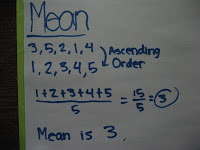### Dean's Measures of Central Tendency

Monday, September 29, 2008
Mean
• Arrange the data in ascending numerical order.
• Add up all the data.
• Divide the sum by how many pieces of data there are.Median
• Arrange the data in ascending numerical order.
• Cross out the border numbers until you remain with one.
• In the occasion of there being 2 numbers, you add them and then divide by 2.
( Sorry for the picture being on its side, i couldn't fix it. )

Mode
• Arrange the data in ascending numerical order.
• Find the most occurring number.
• Note that it is possible to have more than one mode.
( I guess this one is the same as the last picture. )

Range
• Arrange the data in ascending numerical order.
• Subtract the lowest number from the highest number.

Here is a video that explains mean, median and mode :